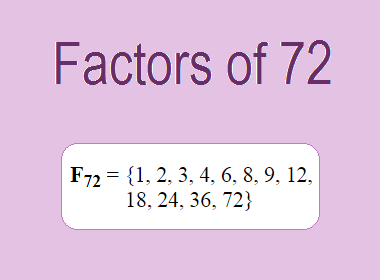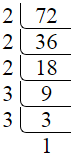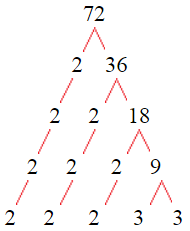# Factors of 72The factors of 72 are 1, 2, 3, 4, 6, 8, 9, 12, 18, 24, 36, and 72 i.e. F72 = {1, 2, 3, 4, 6, 8, 9, 12, 18, 24, 36, 72}. The factors of 72 are all the numbers that can divide 72 without leaving a remainder.

We can check if these numbers are factors of 72 by dividing 72 by each of them. If the result is a whole number, then the number is a factor of 72. Let's do this for each of the numbers listed above:

·        1 is a factor of 72 because 72 divided by 1 is 72.

·        2 is a factor of 72 because 72 divided by 2 is 36.

·        3 is a factor of 72 because 72 divided by 3 is 24.

·        4 is a factor of 72 because 72 divided by 4 is 18.

·        6 is a factor of 72 because 72 divided by 6 is 12.

·        8 is a factor of 72 because 72 divided by 8 is 9.

·        9 is a factor of 72 because 72 divided by 9 is 8.

·        12 is a factor of 72 because 72 divided by 12 is 6.

·        18 is a factor of 72 because 72 divided by 18 is 4.

·        24 is a factor of 72 because 72 divided by 24 is 3.

·        36 is a factor of 72 because 72 divided by 36 is 2.

·        72 is a factor of 72 because 72 divided by 72 is 1.

## How to Find Factors of 72?

1 and the number itself are the factors of every number. So, 1 and 72 are two factors of 72. To find the other factors of 72, we can start by dividing 72 by the numbers between 1 and 72. If we divide 72 by 2, we get a remainder of 0. Therefore, 2 is a factor of 72. If we divide 72 by 3, we get a remainder of 0. Therefore, 3 is also a factor of 72.

Next, we can check if 4 is a factor of 72. If we divide 72 by 4, we get a remainder of 0. Therefore, 4 is also a factor of 72. If we divide 72 by 5, we get a remainder of 2. Therefore, 5 is not a factor of 72. We can continue this process for all the possible factors of 72.

Through this process, we can find that the factors of 72 are 1, 2, 3, 4, 6, 8, 9, 12, 18, 24, 36, and 72. These are the only numbers that can divide 72 without leaving a remainder.

********************

********************

## Properties of the Factors of 72

The factors of 72 have some interesting properties. One of the properties is that the sum of the factors of 72 is equal to 195. We can see this by adding all the factors of 72 together:

1 + 2 + 3 + 4 + 6 + 8 + 9 + 12 + 18 + 24 + 36 + 72 = 195

Another property of the factors of 72 is that the prime factors of 72 are 2, and 3 only.

## Applications of the Factors of 72

The factors of 72 have several applications in mathematics. One of the applications is in finding the highest common factor (HCF) of two or more numbers. The HCF is the largest factor that two or more numbers have in common. For example, to find the HCF of 72 and 80, we need to find the factors of both numbers and identify the largest factor they have in common. The factors of 72 are 1, 2, 3, 4, 6, 8, 9, 12, 18, 24, 36, and 72. The factors of 80 are 1, 2, 4, 5, 8, 10, 16, 20, 40, and 80. The largest factor that they have in common is 8. Therefore, the HCF of 72 and 80 is 8.

Another application of the factors of 72 is in prime factorization. Prime factorization is the process of expressing a number as the product of its prime factors. The prime factors of 72 are 2, and 3 since these are the only prime numbers that can divide 72 without leaving a remainder. Therefore, we can express 72 as:

72 = 2 × 2 × 2 × 3 × 3

We can do prime factorization by division and factor tree method also. Here is the prime factorization of 72 by division method,72 = 2 × 2 × 2 × 3 × 3

Here is the prime factorization of 72 by the factor tree method,72 = 2 × 2 × 2 × 3 × 3

## Conclusion

The factors of 72 are the numbers that can divide 72 without leaving a remainder. The factors of 72 are 1, 2, 3, 4, 6, 8, 9, 12, 18, 24, 36, and 72. The factors of 72 have some interesting properties, such as having a sum of 195. The factors of 72 have several applications in mathematics, such as finding the highest common factor and prime factorization.